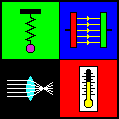# Bohr's Theory of the Hydrogen Atom

In 1913, the Danish physicist Niels Bohr (1885 - 1962) managed to explain the spectrum of atomic hydrogen by an extension of Rutherford's description of the atom. In that model, the negatively charged electrons revolve about the positively charged atomic nucleus because of the attractive electrostatic force according to Coulomb's law.

But the electron can be taken not only as a particle, but also as a de Broglie wave (wave of matter) which interferes with itself. The orbit is only stable, if it meets the condition for a standing wave: The circumference must be an integer multiple of the wavelength. The consequence is that only special values of radius and energy are allowed. The mathematical appendix explains how to calculate these values.

According to classical electrodynamics, a charge, which is subject to centripetal acceleration on a circular orbit, should continuously radiate electromagnetic waves. Thus, because of the loss of energy, the electron should spiral into the nucleus very soon. By contast, an electron in Bohr's model emits no energy, as long as its energy has one of the above-mentioned values. However, an electron which is not in the lowest energy level (n = 1), can make a spontaneous change to a lower state and thereby emit the energy difference in the form of a photon (particle of light). By calculating the wavelengths of the corresponding electromagnetic waves, one will get the same results as by measuring the lines of the hydrogen spectrum.

You must not take the idea of electrons, orbiting around the atomic nucleus, for reality. Bohr's model of the hydrogen atom was only an intermediate step on the way to a precise theory of the atomic structure, which was made possible by quantum mechanics and quantum electrodynamics.

This applet illustrates a hydrogen atom according to particle or wave model. You can choose a principal quantum number n. The right part of the graphics represents the energy levels of the atom. Right down at the bottom you can read off the orbital radius r and the total energy E.

If you try to vary the orbit's radius with pressed mouse button, this will generally lead to a non-stationary state. You can realize that by using the option "Wave model": The green wavy line which symbolizes the de Broglie wave will not be closed in most cases. Only if the circle's circumference is an integer multiple of the wavelength (blue), you will get a stationary state.Physics Applets

URL: http://www.walter-fendt.de/ph14e/bohrh.htm
© Walter Fendt, May 30, 1999
Last modification: January 18, 2003